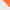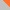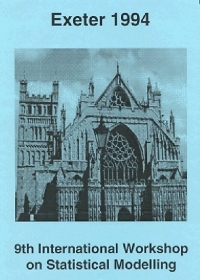Contact# Statistical Modelling Society

www.statmod.orgHomeThe SocietyThe Workshop The IWSM History of the IWSM ArchiveThe JournalNewsletterJoin the SocietyMembers OnlyContact Us

## Ninth International Workshop on Statistical Modelling, Exeter 1994, ProceedingsProceedings of the 9th International Workshop on Statistical Modelling held in Exeter, UK, July 11-15, 1994

## Contents

### Invited and Contributed Papers

R. Davies
Allowing for overdispersion in flow and recurrent choice data
Modelling overdispersion in binomial regression
P Goedhart
The analysis of right-censored count data with and without overdispersion
B Francis
Poisson growth curves, temporal dependence and overdispersion - assessing treatment effects through repeated leaf counts
P Lambert
Modelling of repeated series of count data measured at unequally spaced times
P Smith, J McDonald and J Forster
Monte Carlo exact conditional tests for quasi-independence using Gibbs sampling
J Lang
On likelihood methods for generalized loglinear models
J Van Horebeek and J Teugels
Conditional and marginal independencies in nominal discrete data analysis
N Crichton, J Hinde and J Marchini
Models for diagnosing chest pain: Is CART useful?
J Engel
Some aspects of tree structured curve estimation with Haar-wavelets
K Powell, T Sapatinas, T Bailey and W Krzanowski
Application of wavelets to the pre-processing of underwater sounds
R Little
Modelling the drop-out mechanism in repeated-measures studies
G Verbeke and E Lesaffre
The effect of mis-specifying the random effects distribution in a linear mixed effects model
C Taylor, K Pickering, A Pickles and Sutton
The use of multi-level mixed glms as measurement models in repeated measures experimental data
P Eilers
Sign-constrained , monotone and convex non-parametric regression with asymmetric penalties
R Colombi
A class of log-linear models with parameters subject to non-linear constraints
L Fahrmeir and J Klinger
Estimating and testing generalized linear models under inequality restrictions
Ch Minder and T Bednarski
Robust proportional hazards regression in biomedical applications
GH Oskrochi
A non-parametric frailty model with a parametric and a semi-parametric hazard. An aplication of a counting process and multiplicative intensity model
K Read and M Stasinopoulos
Parametric changepoint models in survival analysis
S Wagenpfeil
Dynamic modelling and posterior mode smoothing in duration and competing risks models
V Dardanoni and A Forcina
A statistical approach to the comparison of mobility tables
M Shoukri
Estimation in mixture quasi-likelihood with power-of-the-mean variance function
G Carmeci and D Gregori
Modelling economic convergence: a GEE approach
W Krzanowski
Some distance measures for ordination of grouped multivariate data
A Baccini, H Caussinus and A de Falguerolles
Diabolic horseshoes
P van der Heijden and J Dessens
Incorporating continuous explanatory variables in latent class analysis
R Hatzinger and W Katzenbeisser
Latent variable models for repeated categorical data
M Green
Social indices and factor analysis for categorical variables
C Payne, G Evans and A Heath
Modelling the class/party relationship in Britain, 1964-92
P Green
Penalised likelihood in non-standard regression problems
G Neubauer and M Schimek
Cross-validated splines and generalized additive models: robustness, scale and variance aspects
B Marx and P Eilers
Direct generalized additive modelling without backfitting
P Royston and D Altman
Improved regression modelling of curved relationships
R Rigby and M Stasinopoulos
An additive model for the dispersion parameter of a generalised model
R Gilchrist and G Portides
Estimating the tuning constant for Huber's M-estimates
P Sebastiani and R Settimi
Planning experiments in non-linear situations
D Firth and J Hinde
D-optimum design for generalized linear and other nonlinear models
W Muller
Optimal design for moving averages
C Vanderhoeft
A decomposition method for fitting non-linear regression models with GLIM
E Ackerley and M Green
IRLS for non-exponential family distributions: model fitting in GLIM4
J Stander
Does Gibbs sampling really help? An example from image analysis
J Mateu and F Montes
Modelling pairwise interaction point processes
D Clayton
Bayesian estimation in generalized linear mixed models with some applications in Biostatistics
B Engel and B Keen
Analysis of glmms with iterated re-weighted REML
R Waterman and B Lindsay
Approximate conditional inference using projected score functions, with examples
N Spencer and R Davies
Consistent parameter estimation for lagged mutlilevel models
H Friedl
Bootstrap procedures in generalized linear models
G Seeber
Computer algebra in statistical modelling : some examples
N Augustin, M Mugglestone and S Buckland
The role of the Gibbs sampler in modeling the spatial distribution of wildlife from incomplete survey data
J Kutylowski
A comparison of strategies for the modelling of ordinal multiresponse data, with application to occupational prestige
D Berridge
Assessing the goodness of fit of regression models for ordinal categorical data

### Posters

J Blake, P Francino and J Francino
Genetic algorithms for time series modelling
E Foumeny, L Bailey and S Afandizadeh
Structural aspects of packed bed design using statistical methods
S Garside and J Whittaker
Stochastic modelling of dynamic systems with SIMULINK
D Hernandez-Rangel
Nonparametric estimation of Markov chains transition functions
M Ka Pui So, K Lam and W Keung Li
The autoregressive random variance model for changing volatility: An alternative to the GARCH model
C Li and W Li
On a double threshold autoregressive heteroskedastic time series model
N Neykov and D Vandev
On the breakdown point properties of LME(k) and LTE(k) in the Gaussian and the Laplace p-th power linear regression
M Ngadiman and A Watkins
Modelling times series transfer functions using the NAG system
A Cohen and M Dagan
Confidence intervals for the between to within variance ratio
P Green
A Bayesian analysis of the multiple change-point problem for a Poisson Process
D Greenhalgh and M Doyle
Detecting replication of individuals in data in which they are identified only by date of birth
P Neytchev
REGRESS PC: A tool for statistical modelling. (Computer demonstration)
R Omar
Multistate models for the analysis of animal carcinogenicity experiments
J Pigeon, J Heyse and H Glick
Modelling the progression of symptomatology associated with benign prostatic hyperplasia
C Schindler, O Brandli and SAPALDIA-Team
Modelling percentiles of lung function variables
E van der Burg, I Noordermeer and H de Haes
Quality of life of cancer patients: a multi-set analysis
R Wilson
Dealing with incomplete data in longitudinal studies
A Amaro
Background modelling units definition. A methodology
V. Barbur and A. Saunders
Practical aspects of principal component analysis in multivariate statistical process control
J Bell
Modelling long-term ecological change in an Oxfordshire woodland
M Cortina-Borja
A comparison of nonparametric correlation tests
D Elston and M Proe
Smoothing regression coefficients in an overspecified regression model with inter-related x-variables
M Green and M Ismail
A semi-parametric method of imputing missing data via surrogate variable in regression model
J Juritz and M Mills
Modelling counts of hyaena populations
S Mejza
A note on the modelling of long-term field experiments
J Stander, A Frigessi and F Divino
Incorporating Geographical Information Systems into Bayesian remote sensing
P Yu and K Lam
Permutation model for duplicate bridge tournament data

© Statistical Modelling Society